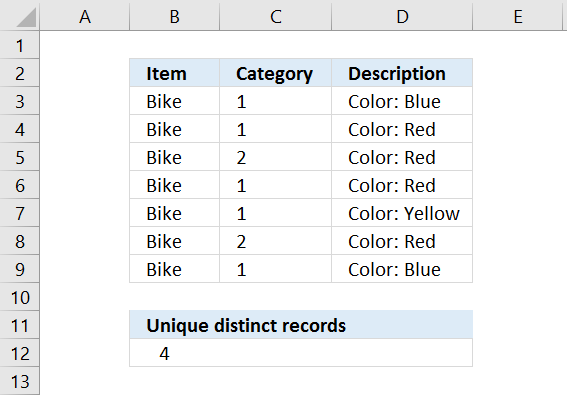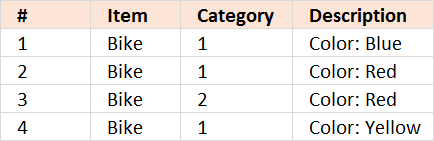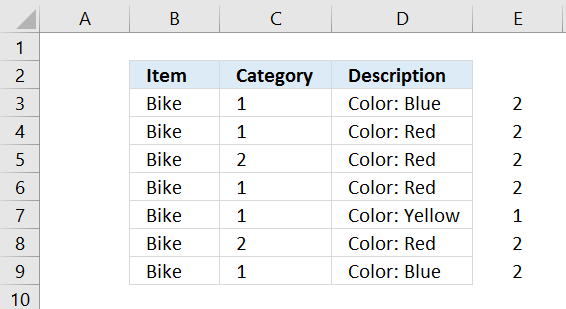Author: Oscar Cronquist Article last updated on January 10, 2019The image above shows a table with 3 columns containing random data. It is quite complicated trying to manually count unique distinct records from this table but Excel can do that for us. A record is an entire row in the table above.

Example, Row 3 has a duplicate in row 9. Row 4 has a duplicate in row 6. Row 5 has a duplicate in row 8. Row 7 is unique meaning there is only one instance of that record in the table. It is also possible to highlight unique distinct records using conditional formatting.

Formula in cell B12:

=SUMPRODUCT(1/COUNTIFS(B3:B9, B3:B9, C3:C9, C3:C9, D3:D9, D3:D9))

#### Watch a video where I explain the formula

https://youtu.be/gDVc5ETknB0

We can verify the count above in cell B12 by extracting all unique distinct records from the above table. I am using a formula from this blog article:
Filter unique distinct row records### Explaining formula in cell B12

=SUMPRODUCT(1/COUNTIFS(C3:C9,C3:C9,D3:D9,D3:D9,E3:E9,E3:E9))

Step 1 - Count each record in data set

COUNTIFS(C3:C9,C3:C9,D3:D9,D3:D9,E3:E9,E3:E9) counts the number of times all criteria match on each row

Example,

The first record is Bike, 1, Color: Blue. The only rows where these criteria match is the first one and last. So the first number in the returning array is 2.I have entered =COUNTIFS(C3:C9,C3:C9,D3:D9,D3:D9,E3:E9,E3:E9) in column E.

COUNTIFS(C3:C9,C3:C9,D3:D9,D3:D9,E3:E9,E3:E9) returns {2;2;2;2;1;2;2}

Step 2 - Divide 1 with array

Why divide 1 with array? If there are two instances of a value the sum returns 1 (0.5 + 0.5 = 1), three instances returns 1 (1/3 + 1/3 + 1/3 = 1) and so on. This lets you count all instances of a value as one.

1/COUNTIFS(C3:C9,C3:C9,D3:D9,D3:D9,E3:E9,E3:E9)

becomes

1/{2;2;2;2;1;2;2}

and returns {0.5;0.5;0.5;0.5;1;0.5;0.5}

Step 3 - Sum values in array

The SUMPRODUCT function lets you sum values, in this case, without entering it as an array formula.

SUMPRODUCT(1/COUNTIFS(C3:C9,C3:C9,D3:D9,D3:D9,E3:E9,E3:E9))

becomes

SUMPRODUCT({0.5;0.5;0.5;0.5;1;0.5;0.5})

and returns 4 in cell range B12

* You will also get a weekly newsletter, unsubscribe anytime!

### Count records with possible blank rows in data setArray formula in cell A28:

=SUMPRODUCT(IFERROR(1/COUNTIFS(\$A\$2:\$A\$25, \$A\$2:\$A\$25, \$B\$2:\$B\$25, \$B\$2:\$B\$25, \$C\$2:\$C\$25, \$C\$2:\$C\$25, \$D\$2:\$D\$25, \$D\$2:\$D\$25), 0))

To enter an array formula, type the formula in a cell then press and hold CTRL + SHIFT simultaneously, now press Enter once. Release all keys.

The formula bar now shows the formula with a beginning and ending curly bracket telling you that you entered the formula successfully. Don't enter the curly brackets yourself.

Count blank rows/records

Formula in B28:

SUMPRODUCT((A2:A25="")*(B2:B25="")*(C2:C25="")*(D2:D25=""))